## CONSTITUTIVE MODELS AND HYPERGRAVITY PHYSICAL SIMULATION OF SOILS$^{\bf 1)}$

Chen Yunmin,2), Ma Pengcheng, Tang Yao

Center for Hypergravity Experimental and Interdisciplinary Research, Zhejiang University, Hangzhou 310058, China

 基金资助: 1)国家自然科学基金.  51988101

Received: 2019-03-2   Accepted: 2020-04-14   Online: 2020-07-18Abstract

Numerical simulation and physical simulation are two main methods to analyze the settlement and stability of soil mass. As the mathematical equations of the soil stress-strain relationships, constitutive models are the foundations of numerical simulation. Soil is a type of granular materials, leading to three essential characteristics of it including compressive hardening, shear dilatancy and friction. They are the main characteristics differing soils from metals and should be considered in constitutive model of soils. Traditional soil mechanics, which are widely applied in engineering at present, analyze the deformation and strength of soils separately by elastic theory and limit equilibrium theory based on rigid plasticity, respectively. However, the accuracy of their calculation results is generally difficult to satisfy the requirement of quantitative analysis because the essential characteristics of soils cannot be fully reflected. Cam-clay model is the first elasto-plastic constitutive model that can fully reflect the essential characteristics of soils. It unifies the deformation and strength of soils and can well describe the stress-strain relationships of normal consolidated clays; thus, Cam-clay model is regarded as the beginning of modern soil mechanics. Through introducing a unique unified hardening parameter, unified hardening model further develops the Cam-clay model and enlarges the application scope to over-consolidated clays. The authors believe that the challenge of constitutive model research in the future is how to consider the phase change of soil skeleton and the multi-field coupling in soils, to solve significant geotechnical problems in the field of energy, traffic, environment and hydraulic engineering, which cannot be analyzed quantitatively by current models. Due to the effects of scale compression and time compression, hypergravity physical simulation can overcome the disadvantage that stress level in small-scale model is lower than the prototype level in normal gravity physical simulation. Especially, hypergravity physical simulation is very appropriate to the problems of large scale and long duration. Compared with numerical simulation, hypergravity physical simulation has the advantages of being able to test the rationality of soil constitutive models and reveal the unknown features that cannot be described by current models. Finally, an engineering case of large-diameter steel pipe pile analyzed by combining numerical simulation and hypergravity physical simulation was presented.

Keywords： soil ; numerical simulation ; physical simulation ; constitutive model ; hypergravity

Chen Yunmin, Ma Pengcheng, Tang Yao. CONSTITUTIVE MODELS AND HYPERGRAVITY PHYSICAL SIMULATION OF SOILS$^{\bf 1)}$. Chinese Journal of Theoretical and Applied Mechanics[J], 2020, 52(4): 901-915 DOI:10.6052/0459-1879-20-059

## 引言

### 图1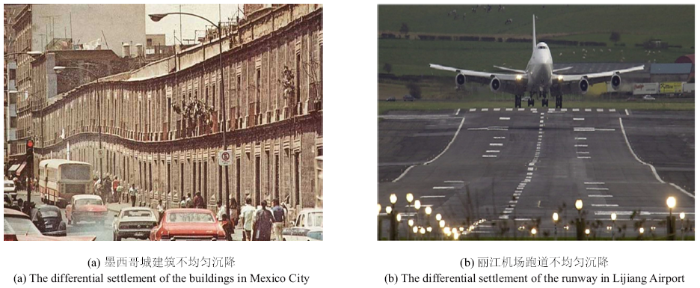Fig. 1   The effect on normal use of structure caused by the differential settlement of soils

### 图2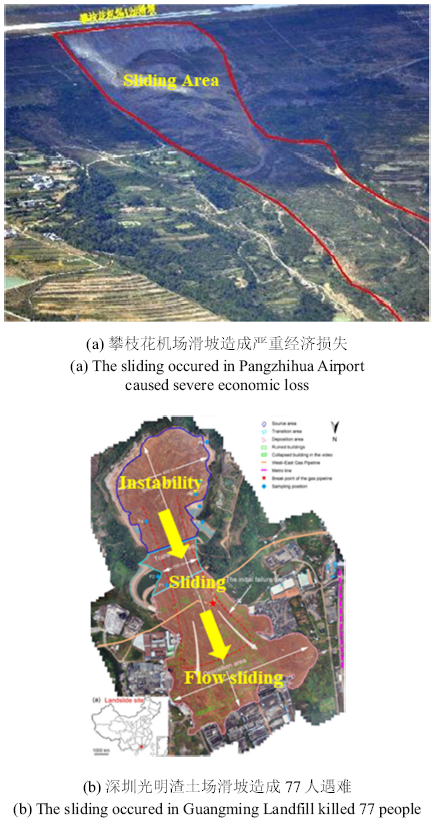Fig. 2   The slope sliding caused by the shear failure of soils

## 1 土的基本特性

(1)压硬性是土的变形模量随着均应力的增大而增大的特性. 以等向压缩试验为例, 其均应力-体应变($p-\varepsilon_{\rm v})$关系的示意图见图3(a), 随着均应力的增大, 土产生体积变形, 孔隙体积缩小, 变形中绝大部分是不可恢复的塑性变形; 随着土变得越来越致密, 其体积变形模量明显增大, 表现出"越压越硬"的特点, 体现出了土的压硬性. 然而, 金属材料的应力应变关系则明显不同. 金属的内部晶格之间的排列非常紧密, 在等向压力下不会产生晶格间的滑移, 因此产生的塑性体应变非常小.

### 图3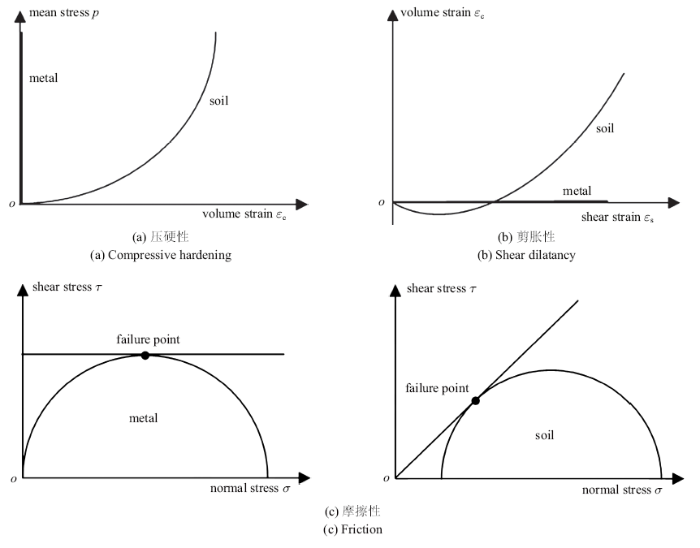Fig. 3   The essential characteristics of soils

(2)剪胀性是土在剪应力作用下体积发生变化的特性, 一般将体积膨胀和收缩统称为剪胀性. 纯剪切作用下土和金属的体应变-剪应变($\varepsilon_{\rm v}$-$\varepsilon_{\rm s})$关系的示意图见图3(b), 可见, 土在纯剪切作用下产生了明显的体积应变和剪应变, 而金属仅产生剪应变. 土的剪胀性是由剪切过程中土骨架变形、土颗粒相对位置变化导致的, 对于比较松散的土(如正常固结土和松砂), 土颗粒移动后会填充到原先的孔隙中, 使其排列变得更为紧密, 在宏观上表现为体积的缩小; 对于比较致密的土(如超固结土和密砂), 原本排列紧密的土颗粒在发生移动后会产生更大的孔隙, 使其排列变得更为松散, 在宏观上表现为体积的膨胀. 金属材料在剪切作用下只发生晶格的相对滑移, 不产生体积变形.

(3)摩擦性是土的剪切强度随剪切面上正应力的增大而增大的特性. 如图3(c)所示, Tresca准则是判别金属材料屈服和破坏的常用判据, 即破坏面是最大剪应力面, 且剪切强度不会因破坏面上的正应力的变化而变化; 然而, 土的剪切破坏通常使用Mohr-Coulomb准则判别, 剪切破坏发生在剪应力和正应力之比最大的面上. 因此, 随着破坏面上正应力的增大, 剪切强度也会相应增大, 这体现了土的摩擦性. 土是颗粒材料, 剪切破坏时需要克服破坏面上土颗粒间的摩擦力, 这是剪切强度与正应力成正比的根本原因, 这同时也表明碎散性的基本物理特性对土体力学特性的决定作用.

### 图4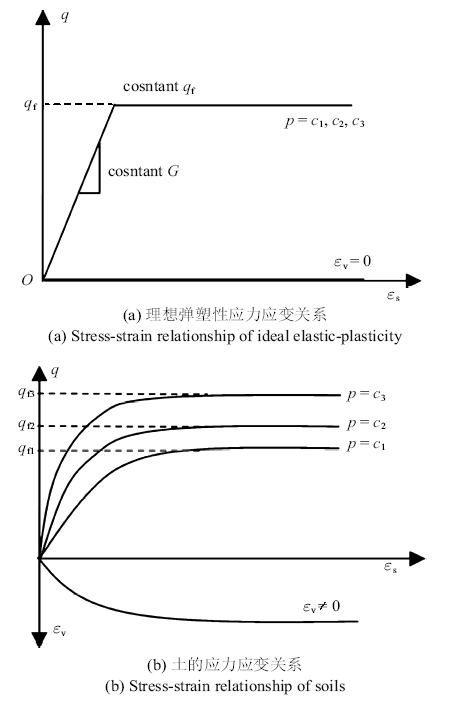Fig. 4   The differences between the stress-strain relationships of ideal elastic-plasticity and soils

## 2 土的本构模型

### 2.1 传统土力学变形和强度理论

1923年, Terzaghi提出了土的有效应力和一维固结理论的概念, 标志着土力学成为了一门独立的学科. 由Terzaghi主导建立的, 以有效应力原理、基于弹性力学的变形分析理论和基于刚塑性模型的破坏分析理论为主要框架的土力学称为传统土力学或古典土力学.

### 图5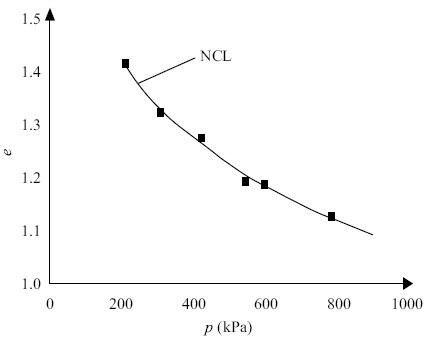Fig. 5   The normal compression line (NCL) of normally consolidated clay

### 2.2 剑桥模型(Cam-clay model)

2.2.1 正常固结土的力学特性

(1)压硬性

\begin{equation} e=N-\lambda \ln p \end{equation}

\begin{equation} \varepsilon _{\rm v} =\frac{\lambda }{1+e_0 }\ln \frac{p}{p_0 } \end{equation}

\begin{equation} \varepsilon _{\rm v}^{\rm e} =\frac{\kappa }{1+e_0 }\ln \frac{p}{p_0 } \end{equation}

\begin{equation} \varepsilon _{\rm v}^{\rm p} =\frac{\lambda -\kappa }{1+e_0 }\ln \frac{p}{p_0 }=c_{\rm p} \ln \frac{p}{p_0 } \end{equation}

### 图6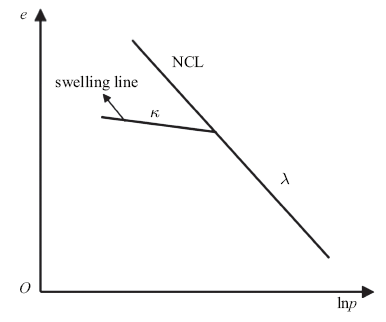Fig. 6   NCL and swelling line of normally consolidated clay

(2)摩擦性

\begin{equation} q_{\rm f} =Mp \end{equation}

### 图7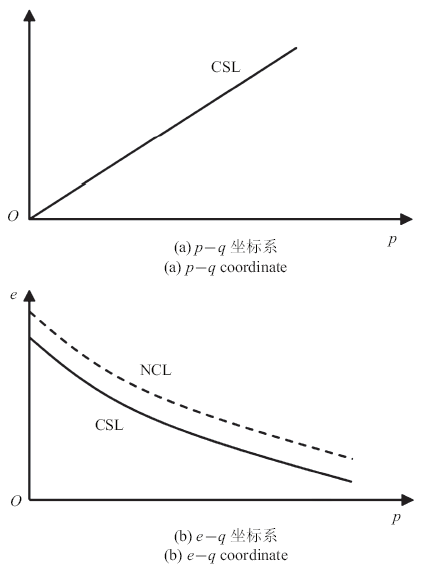Fig. 7   Critical state line (CSL) of normally consolidated clay

(3)剪胀性

\begin{equation} \frac{{\rm d}\varepsilon _{\rm v}^{\rm p}}{{\rm d}\varepsilon _{\rm s}^{\rm p} }=f\left( {\eta ,C} \right) \end{equation}

Roscoe和Burland基于能量方程推导了修正模型所采用的剪胀方程. 土单元体塑性功的一般表达式为

\begin{equation} {\rm d}W^{\rm P}=p{\rm d}\varepsilon _{\rm v}^{\rm p} +q{\rm d}\varepsilon _{\rm s}^{\rm p} \end{equation}

\begin{equation} \left.\begin{array}{l} {\rm d}W_{\rm A}^{\rm P} =Mp{\rm d}\varepsilon _{\rm s}^{\rm p} \\ {\rm d}W_{\rm B}^{\rm P} =p{\rm d}\varepsilon _{\rm v}^{\rm p} \\ \end{array}\right\} \end{equation}

\begin{equation} {\rm d}W^{\rm P}=p\sqrt {\left( {{\rm d}\varepsilon _{\rm v}^{\rm p} } \right)^2+\left( {M{\rm d}\varepsilon _{\rm s}^{\rm p} } \right)^2} \end{equation}

\begin{equation} \frac{{\rm d}\varepsilon _{\rm v}^{\rm p} }{{\rm d}\varepsilon _{\rm s}^{\rm p} }=\frac{M^2-\eta ^2}{2\eta } \end{equation}

### 图8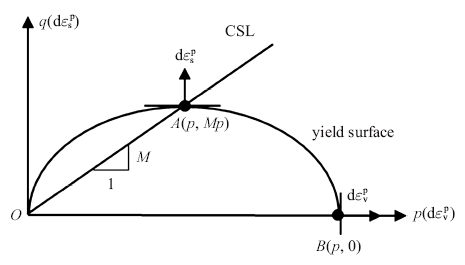Fig. 8   Schematic diagram of the yield surface of Cam-clay model

2.2.2 修正剑桥模型的屈服面和硬化规律

\begin{equation}f\left( {\sigma _{ij} ,H} \right)=0\end{equation}

\begin{equation}{\rm d}p{\rm d}\varepsilon _{\rm v}^{\rm p} +{\rm d}q{\rm d}\varepsilon _{\rm s}^{\rm p} =0\end{equation}

\begin{equation}\frac{{\rm d}p}{{\rm d}q}+\frac{M^2p^2-q^2}{2pq}=0\end{equation}

\begin{equation}q^2+M^2p^2-M^2p_x p=0\end{equation}

\begin{equation}g=f=\ln \frac{p}{p_0 }+\ln \left( {1+\frac{q^2}{M^2p^2}} \right)-\frac{1}{c_{\rm p} }\varepsilon _{\rm v}^{\rm p} =0\end{equation}

2.3.1 超固结土的力学特性

### 图9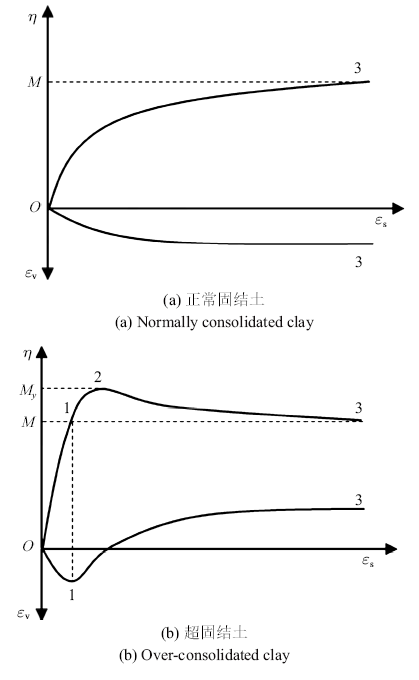Fig. 9   Stress-strain relationships in triaxial tests of normally and over consolidated clay

(1)压硬性

\begin{equation}{\rm d}\varepsilon _{\rm v}^{\rm p} =\frac{\bar{{\lambda }}-\kappa }{1+e_0 }\frac{{\rm d}p}{p}\end{equation}

### 图10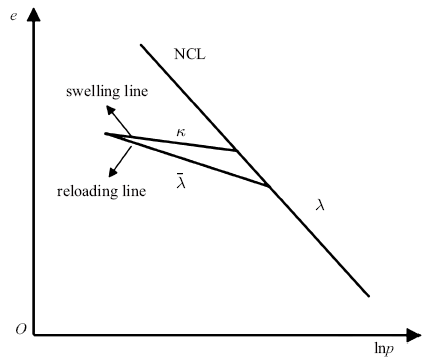(2)摩擦性

\begin{equation}M_{\rm f} =\left( {\frac{1}{R_0 }-1} \right)\left( {M-M_{\rm h} } \right)+M\end{equation}

### 图11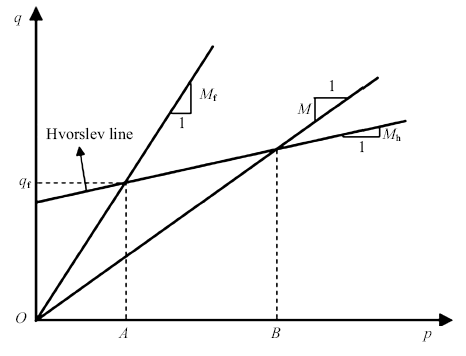(3)剪胀性

UH模型仍然采用修正剑桥模型的剪胀方程(式(10)). 如前文所述, 土的剪胀方程决定屈服面的形状, 因此UH模型的屈服面也可用式(14)描述. 但是, 与正常固结土不同的是, 超固结土在硬化阶段即出现先剪缩后剪胀现象, 也就是说, 在$p_{x}$增大时$\varepsilon _{\rm v}^{\rm p}$产生了非单调的变化, 因此$p_{x}$和$\varepsilon _{\rm v}^{\rm p}$不是唯一对应的, 不能继续采用$\varepsilon _{\rm v}^{\rm p}$作为硬化参数.

2.3.2 统一硬化模型的屈服面和硬化规律

\begin{equation}f=\ln \frac{p}{p_0 }+\ln \left( {1+\frac{q^2}{M^2p^2}} \right)-H=0\end{equation}

\begin{equation}f=\ln \frac{\bar{{p}}}{\bar{{p}}_0 }+\ln \left( {1+\frac{\bar{{q}}^2}{M^2\bar{{p}}^2}} \right)-\frac{1}{c_{\rm p} }\varepsilon _{\rm v}^{\rm p} =0\end{equation}

\begin{equation}R=\frac{p}{\bar{{p}}}\end{equation}

\begin{equation}R=\frac{p}{\bar{{p}}_0 }\left( {1+\frac{q^2}{M^2p^2}} \right)\exp \left({-\frac{\varepsilon _{\rm v}^{\rm p} }{c_{\rm p} }} \right)\end{equation}

\begin{equation}M_{y} =\left( {\frac{1}{R}-1} \right)\left( {M-M_{\rm h} } \right)+M\end{equation}

### 图12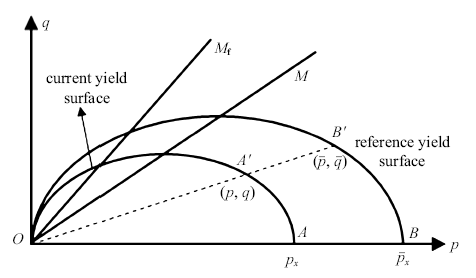Fig. 12   Current and reference yield surfaces

\begin{equation}H=\frac{1}{c_{\rm p} }\int {\frac{M_{\rm y}^4 -\eta ^4}{M^4-\eta ^4}}{\rm d}\varepsilon _{\rm v}^{\rm p}\end{equation}

(a) $O\to 1$阶段, $0<\eta \leqslant M$, 特点是剪缩、硬化, 点1 ($\eta=M)$为剪缩和剪胀的分界点(又称为特征点). 根据式(18), UH模型椭圆屈服面顶点的应力比为$M$, 当应力比低于$M$时, 根据正交流动法则可判断为剪缩变形, 故采用UH模型屈服面可以描述特征点的应力比和此阶段的剪缩变形; 在此应力比范围内, d$H>0$, 故采用统一硬化参数可以描述此阶段的硬化.

(b) $1\to 2$阶段, $M<\eta\leqslant M_{y}$(此处$M_{y}$为2点的当前强度), 特点是剪胀、硬化, 点2 ($\eta =M_{y})$为硬化和软化的分界点. 当应力比大于$M$时, 根据正交流动法则, 采用UH模型屈服面可以描述该阶段的剪胀变形; 在此应力比范围内, d$H>0$, 故采用统一硬化参数可以描述此阶段的硬化.

(c) $2\to 3$阶段, $\eta\geqslant M_{y}\geqslant M$, 特点是剪胀、软化, 在此阶段当前强度降低, 应力比也随之降低但略大于当前强度; 最终达到临界状态, 应力比和当前强度均降低至$M$ ($\eta =M_{y}=M$, 点3). 显然, 采用UH模型屈服面可以描述该阶段的剪胀变形; 在此应力比范围内, d$H<0$, 故采用统一硬化参数可以描述此阶段的软化.

### 图13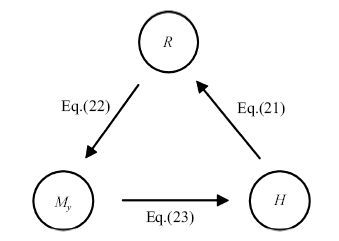Fig. 13   The relationship among $R$, $M_{y}$ and $H$

## 3 土体的超重力物理模拟

### 图14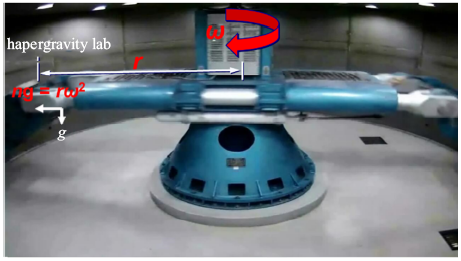Fig. 14   ZJU-400 centrifuge

(1)缩尺效应

### 图15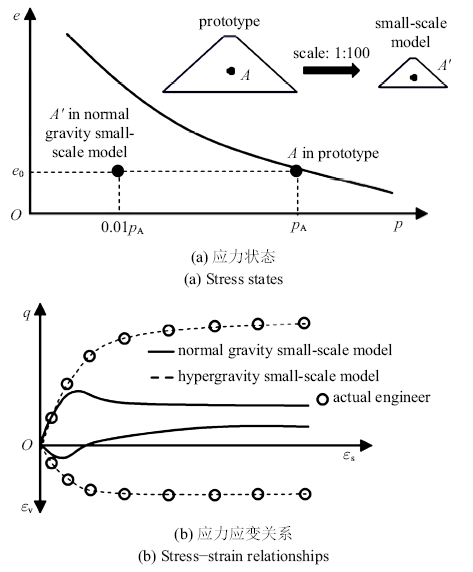Fig. 15   Schematic diagram of the comparison between small-scale model and prototype

(2)缩时效应

## 4 案例——大直径钢管桩水平受荷特性

### 图16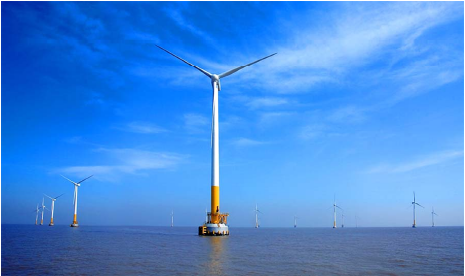Fig. 16   Single pile foundation of offshore wind turbine

Table 1  Parameters of UH model### 图17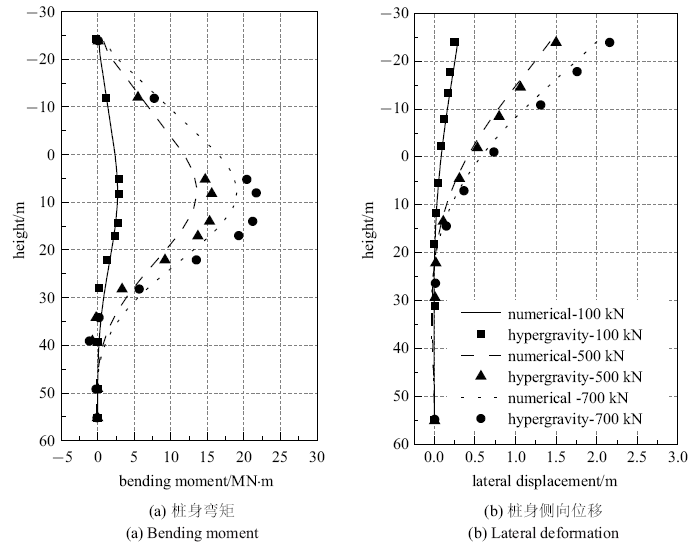Fig. 17   Results of hypergravity physical and numerical simulations of the single pile foundation of offshore wind turbine

## 5 结论与展望

(1)土是碎散的工程材料, 具有压硬性、摩擦性和剪胀性, 这是土的工程力学特性区别于金属的主要特征.

(2)土体的本构模型发展先后历经了强度和变形分离考虑的传统土力学阶段和以剑桥模型、统一硬化模型为代表的全面反映压缩性、摩擦性和剪胀性的现代土力学阶段, 对土体复杂应力应变关系的描述效果有明显的提高. 未来本构模型的发展趋势为考虑岩土体在受力过程中土骨架相变和多场耦合, 旨在为目前本构模型无法定量分析的能源、交通、环境和水利相关的重大岩土工程问题提供合适的数值分析工具.

(3)超重力物理模拟具有缩尺和缩时效应, 不仅可验证土体本构模型的合理性, 还可以探索现有本构模型无法描述的土体未知特性, 在工程中可与数值模拟联合应用, 相互验证. 除岩土外, 超重力物理模拟还可应用于地质、资源和材料等重要领域. 国家重大科技基础设施超重力离心模拟与实验装置作为综合超重力多领域学科实验平台, 建成后将全面应用于上述领域.

## 参考文献 原文顺序 文献年度倒序 文中引用次数倒序 被引期刊影响因子

Zhan LT, Zhang Z, Chen YM, et al.

The 2015 Shenzhen catastrophic landslide in a construction waste dump: Reconstitution of dump structure and failure mechanisms via geotechnical investigations

Engineering Geology, 2018,238:15-26

UH模型系列研究

URL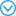( Yao Yangping.

Chinese Journal of Geotechnical Engineering, 2016,37(2):193-217 (in Chinese))

URL( Yao Yangping, Zhang Binyin, Zhu Jungao.

Behaviors, constitutive models and numerical simulation of soils

China Civil Engineering Journal, 2012(3):135-158 (in Chinese))

( Terzaghi K. Theoretical Soil Mechanics. Xu ZY translated. Beijing: Geological Publishing House, 1960 (in Chinese))

Roscoe KH, Schofield AN, Thurairajah A.

Yielding of clays in states wetter than critical

Géotechnique, 1963,13(3):211-240

Roscoe KH, Burland JB. On the Generalized Stress-Strain Behaviour of Wet Clay. Engineering Plasticity, Cambridge: Cambridge University Press, 1968: 535-609

( Li Guangxin. Advanced Soil Mechanics. Beijing: Tsinghua University Press, 2004 (in Chinese))

URLConstitutive modeling theory for soils is very significant in geotechnical engineering. The Cam - Clay elasto - plastic model is widely regarded as the milestone of modern soil mechanics. The concept of the model has explicit geometric and physical meanings. The Cam - Clay model and its modified model are regarded as one of several well accepted models among many proposed models. The improvement and revision based on the Cam - Clay model are the important aspect of modeling for geomaterial. In this paper, the Cam - Clay model and its modified model are introduced in brief, its limitation i
s analysed and an overview on the improvement of this model is given to farther
study. 

( Xu Shunhua, Xu Guangli, Zhang Li.

Review of Cam-clay model for soils

Journal of Yangtze River Scientific Research Institute, 2007,24(3):27-32 (in Chinese))

URLConstitutive modeling theory for soils is very significant in geotechnical engineering. The Cam - Clay elasto - plastic model is widely regarded as the milestone of modern soil mechanics. The concept of the model has explicit geometric and physical meanings. The Cam - Clay model and its modified model are regarded as one of several well accepted models among many proposed models. The improvement and revision based on the Cam - Clay model are the important aspect of modeling for geomaterial. In this paper, the Cam - Clay model and its modified model are introduced in brief, its limitation i
s analysed and an overview on the improvement of this model is given to farther
study. 

Nakai T, Matsuoka H.

A generalized elastoplastic constitutive model for clay in three-dimensional stresses

Soils and Foundations, 1986,26(3):81-98

Newson TA.

Validation of a non-associated critical state model

Computers and Geotechnics, 1998,23(4):277-287

Yin JH, Graham J.

Elastic viscoplastic modelling of the time-dependent stress-strain behaviour of soils

Yao YP, Sun DA, Matsuoka H.

A unified constitutive model for both clay and sand with hardening parameter independent on stress path

Computers and Geotechnics, 2008,35(2):210-222

URL## Abstract

A unified constitutive model for both clay and sand under three-dimensional stress conditions is derived from the modified Cam-clay model, by taking the following two points into consideration. First, a transformed stress tensor based on the SMP (spatially mobilized plane) criterion is applied to the Cam-clay model. The proposed model consistently describes shear yielding and shear failure and combines critical state theory with the SMP criterion for clay. Secondly, a new hardening parameter, which is independent of the stress path, is derived in order to develop a unified constitutive model for both clay and sand. It not only describes the dilatancy for lightly to heavily dilatant sand, but also reduces to the plastic volumetric strain for clay. The validity of the hardening parameter is confirmed by the test results of triaxial compression and extension tests on sand under various stress paths. Only five conventional soil parameters are needed in the proposed model.

Yao YP, Hou W, Zhou AN.

UH model: Three-dimensional unified hardening model for overconsolidated clays

Géotechnique, 2009,59:451-469

[博士论文]. 哈尔滨: 中国地震局工程力学研究所, 2019

( Wang Hai.

Research on several crucial problems of geotechnical centrifuge modelling techniques

[PhD Thesis]. Haerbin: Institute of Engineering Mechanics,China Earthquake Administration, 2019 (in Chinese))

Craig WH.

édouard Phillips (1821-89) and the idea of centrifuge modelling

Géotechnique, 1989,39(4):697-700

Pokrovsky GI.

Studies of soil pressures and soil deformations by means of a centrifuge

// Proceedings of the 1st ICSMFE, 1936,1:70-71

( Wang Zhen.

Simulate the millennium in one day——Zhejiang University leads the construction of the world's largest hypergravity centrifugal simulation and experimental device

China Consulting Engineers, 2019(2):93-94 (in Chinese))

Roscoe KH, Schofield AN.

Mechanical behaviour of an idealised 'wet clay'//Proceedings of the 3rd European Conference on Soil Mechanics and Foundation Engineering

( Shen Zhujiang. Theoretical Soil Mechanics Beijing: China Water&Power Press, 2000 (in Chinese))

Biot MA.

General Theory of three-Dimensional consolidation

Journal of Applied Physics, 1941,12(2):155

Duncan JM.

Limitations of conventional analysis of consolidation settlement

Journal of Geotechnical Engineering, 1993,119(9):1333-1359

Davis EH, Raymond GP.

A non-linear theory of consolidation

Géotechnique, 1965,15(2):161-173

Schofield A, Wroth P.

Critical State Soil Mechanics

London: McGraw-Hill, 1968

Rowe PW.

The stress-dilatancy relation for static equilibrium of an assembly of particles in contact//Proceedings of the Royal Society of London. Series A

Mathematical and Physical Sciences, 1962,269(1339):500-527

Li XS, Dafalias YF.

Dilatancy for cohesionless soils

Géotechnique, 2000,50(4):449-460

Mita KA, Dasari GR, Lo KW.

Performance of a three-dimensional Hvorslev-modified Cam clay model for overconsolidated clay

International Journal of Geomechanics, 2004,4(4):296-309

URLFor soils, the variations of the mean stress and the deviatoric stress induce plastic volume strain and plastic shearing strain. Based on the dilatancy equation, a coupling stress is proposed considering the influences of both the mean stress and the deviatoric stress on plastic strain. With the isotropic compression and swelling regularities of clay, referring to the necessary and sufficient conditions of path independency for curvilinear integration, a one-dimensional constitutive model describing the relationship between the plastic volume strain and the coupling stress is deduced. By using this method, an elasto-plastic constitutive relationship for sand is also established. From a new visual angle, a method for understanding and establishing the soil constitutive model is introduced by deducing the coupling stress and one-dimensional constitutive relation.

( Yao Yangping, Zhu Enyang.

Establishing soil constitutive model based on coupling stress

Chinese Journal of Geotechnical Engineering, 2010,32(12):1922-1929 (in Chinese))

URLFor soils, the variations of the mean stress and the deviatoric stress induce plastic volume strain and plastic shearing strain. Based on the dilatancy equation, a coupling stress is proposed considering the influences of both the mean stress and the deviatoric stress on plastic strain. With the isotropic compression and swelling regularities of clay, referring to the necessary and sufficient conditions of path independency for curvilinear integration, a one-dimensional constitutive model describing the relationship between the plastic volume strain and the coupling stress is deduced. By using this method, an elasto-plastic constitutive relationship for sand is also established. From a new visual angle, a method for understanding and establishing the soil constitutive model is introduced by deducing the coupling stress and one-dimensional constitutive relation.

Nakai T, Hinokio M.

A simple elastoplastic model for normally and over consolidated soils with unified material parameters

Soils and Foundations, 2004,44(2):53-70

Klar A, Soga K, Ng MYA.

Coupled deformation-flow analysis for methane hydrate extraction

Geotechnique, 2010,60(10):765-776

Arenillas A, Pevida C, Rubiera F.

Comparison between the reactivity of coal and synthetic coal model

Fuel, 2003,82(15):2001-2006

Chen YM, Xu WJ, Ling DS, et al.

A degradation-consolidation model for the stabilization behavior of landfilled municipal solid waste

Computers and Geotechnics, 2020,118:103341

Sun YF, Xiao Y, Ju W.

Bounding surface model for ballast with additional attention on the evolution of particle size distribution

Science China Technological Sciences, 2014,57(7):1352-1360

( Zhu Sheng, Zhong Chunxin, Zheng Xilei, et al.

Filling standards and gradation optimization of rockfill materials

Chinese Journal of Geotechnical Engineering, 2018,40(1):114-121 (in Chinese))

Mcdowell GR, Bolton MD, Robertson D.

The fractal crushing of granular materials

Journal of the Mechanics and Physics of Solids, 1996,44(12):2079-2102

[博士论文]. 杭州: 浙江大学, 2015

( Zeng Xing.

Similitude for centrifuge modelling of heavy metal migration in clay barrier and method for evaluating breakthrough time

[PhD Thesis]. Zhejiang University, 2015 (in Chinese))

Zhou YG, Chen YM.

Laboratory investigation on assessing liquefaction resistance of sandy soils by shear wave velocity

Journal of Geotechnical and Geoenvironmental Engineering, 2007,133(8):959-972

Kayen R, Moss RES, Thompson EM, et al.

Shear-wave velocity-based probabilistic and deterministic assessment of seismic soil liquefaction potential

Journal of Geotechnical and Geoenvironmental Engineering, 2013,139(3):407-419

Zhu B, Qian H, Kong D, et al.

Field trials on monotonic behaviour of a driven pile at an offshore wind farm in China

Marine Georesources & Geotechnology, 2019: 1-11

Hu HJE, Leung CF, Chow YK, et al.

Centrifuge modelling of SCR vertical motion at touchdown zone

Ocean Engineering, 2011,38(7):888-899

URL## Abstract

Steel catenary risers (SCR) connect seabed pipelines and flow lines to floating structures used for oil and gas production in deep waters. Waves and currents induce motions of the structure and the risers. The repeated motions of the risers at the touchdown zone in turn induce loads on the seabed soil and might eventually cause fatigue damage to the risers. The analysis of riser fatigue damage is heavily dependent on the soil model. Soil behaviour at touchdown zone such as soil remoulding, stiffness degradation and deformation of the seabed at the touchdown zone further complicate the accurate assessment of riser fatigue damage, which is currently not appropriately quantified in existing design methods. This paper presents centrifuge model tests simulating the repeated vertical movement of a length of riser on clay seabed with increasing undrained shear strength with depth. During the tests, the pipe was subject to cyclic motion over fixed vertical displacement amplitude from an invert embedment of 0.5–3.5 pipe diameters into the soil. The test results show a significant progressive degradation of soil strength and diminution of excess pore water pressure with increasing number of riser penetration/uplift cycle. In view of the different types of environment loadings experienced by floating platforms and various soil conditions, tests were also conducted to investigate the effect of soil strength, riser displacement rate and loading mode on riser–soil interaction during repetitive penetration/uplift motion of the riser.

/

 〈〉